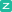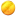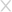## 简明手册：如何在PHP应用中防止SQL注入2015-05-29+5 373101人围观 ，发现 7 个不明物体

SQL注入是一个控制数据库查询的技术，往往会导致丧失机密性。在某些情况下，如果成功执行

SELECT '<?php eval(base64_decode("someBase64EncodedDataHere"));' INTO OUTFILE '/var/www/reverse_shell.php'

$stmt =$pdo->prepare('SELECT * FROM blog_posts WHERE YEAR(created) = ? AND MONTH(created) = ?');if (!$stmt->execute([$_GET['year'], $_GET['month']])) { header("Location: /blog/"); exit; }$posts = $stmt->fetchAll(\PDO::FETCH_ASSOC); 在PHP应用中预处理语句过滤掉任何SQL注入的可能，无论是什么都需要先传递到$_GET变量。SQL查询语句是攻击者无法改变的（除非你将PDO::ATTR_EMULATE_PREPARES 开启了，这也意味着你还没有真正使用预处理语句）XKCD作者Randall Munroe是个聪明人。如果这幅漫画直到今天才被创作出来，大概会是这个样子的$email = filter_var($_POST['email'], FILTER_VALIDATE_EMAIL);
if (empty($email)) { throw new \InvalidArgumentException('Invalid email address'); } 列和表标识符 当列和表标识符作为查询语句中的一部分，你不能使用参数表示它们。如果你正在开发的这个应用需要一个动态查询结构，请使用白名单。 白名单是一个应用程序逻辑策略，它只允许少数可信的值。相对来说黑名单，仅仅是禁止已知的恶意输入。 大多数情况下，使用白名单比黑名单更安全！ $qs = 'SELECT * FROM photos WHERE album = ?';
// Use switch-case for an explicit whitelist
switch ($_POST['orderby']) { case 'name': case 'exifdate': case 'uploaded': // These strings are trusted and expected$qs .= ' ORDER BY '.$_POST['orderby']; if (!empty($_POST['asc'])) {
$qs .= ' ASC'; } else {$qs .= ' DESC';
}
default:
// Some other value was passed. Let's just order by photo ID in descending order.
$qs .= ' ORDER BY photoid DESC'; }$stmt = $db->prepare($qs)
;if ($stmt->execute([$_POST['album_id']])) {
$photos =$stmt->fetchAll(\PDO::FETCH_ASSOC);
}

1. 你可以使用EasyDB包含你的PDO

2. 如果你熟悉PDO构造，你可以使用\ParagonIE\EasyDB\Factory::create()参数来进行替代

// First method:
$pdo = new \PDO('mysql;host=localhost;dbname=something', 'username', 'putastrongpasswordhere');$db = \ParagonIE\EasyDB\EasyDB($pdo, 'mysql'); // Second method:$db = \ParagonIE\EasyDB\Factory::create('mysql;host=localhost;dbname=something', 'username', 'putastrongpasswordhere');

$data =$db->safeQuery(
'SELECT * FROM transactions WHERE type = ? AND amount >= ? AND date >= ?',
[
$_POST['ttype'],$_POST['minimum'],
$_POST['since'] ] ); 从一个数据库表中选择多行 $rows = $db->run( 'SELECT * FROM comments WHERE blogpostid = ? ORDER BY created ASC',$_GET['blogpostid']
);
foreach ($rows as$row) {
$template_engine->render('comment',$row);
}

$userData =$db->row(
"SELECT * FROM users WHERE userid = ?",
$_GET['userid'] ); 向数据库表中插入新的一行 $db->insert('comments', [
'blogpostid' => $_POST['blogpost'], 'userid' =>$_SESSION['user'],
'comment' => $_POST['body'], 'parent' => isset($_POST['replyTo']) ? $_POST['replyTo'] : null ]); 动态查询中躲避标识符（列/表/视图名） $qs = 'SELECT * FROM some_table';
$and = false; if (!empty($where)) {
$qs .= ' WHERE '; foreach (\array_keys($where) as $column) { if (!\in_array($column, $whiteListOfColumnNames)) { continue; } if ($and) {
$qs .= ' AND '; }$qs .= $db->escapeIdentifier($column).' = ?';
$and = true; } }$qs .= ' ORDER BY rowid DESC';
// And then to fetch some data
$data =$db->run($qs, \array_values($where);

*参考来源github，译者/鸢尾 转载请注明来自FreeBuf黑客与极客（FreeBuf.COM）### 已有 7 条评论

• (1级)  2015-05-29 回复 1楼

抢！

• (1级)  2015-05-29 回复 2楼

参考来源github?
有連結嗎~大大

• (2级)  2015-05-30 回复

@ rockway  https://paragonie.com/blog/2015/05/preventing-sql-injection-in-php-applications-easy-and-definitive-guide

•过路人  2015-05-29 回复 3楼

英语六级的路过

• (4级)  2015-05-29 回复 4楼

= =。我一般用medoo

• (5级) AMD®Zen.Team.com.org.cn.net/lo...  2015-05-29 回复 5楼

为毛不是中文漫画?zhe shi shen me gui manhua!!!!!!!wan quan 看不懂!!!

•momoda  2015-05-31 回复 6楼

大声的告诉我这个彩笔～实体框架是不是都是用的参数化查询### 相关阅读

推荐关注官方公众号聚焦企业安全### 填写个人信息

• 可以给我们打个分吗？